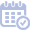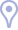Talks

By YearBy Topic

Statistical Estimation

Exploiting Statistical Dependencies in Sparse RepresentationsJanuary 7th, 2011Invited talk in the SMALL workshop on Sparse Dictionary Learning.

In the commonly used sparse representation modeling, the atoms are assumed to be independent of each other when forming the signal. In this talk we shall introduce a statistical model called Boltzman Machine (BM) that enables such dependencies to be taken into account. Adopting a Bayesian point of view, we first treat the pursuit problem – given a signal, and assuming that the model parameters and the dictionary are known, find its sparse representation. We derive the exact MAP estimation, and show that just like in the independent case, this leads to an exponential search problem. We derive two algorithms for its evaluation: a greedy approximation approach for the general case, and an exact estimation that corresponds to a unitary dictionary and banded interaction matrix. We also consider the estimation of the model parameters, learning these parameters directly from training data. We show that given the signals’ representations, this problem can be posed as a convex optimization task by using the Maximum Pseudo-Likelihood (MPL).This is a joint work with Tomer Faktor and Yonina Eldar (EE - Technion).
Topics in Minimum-Mean-Squared-Error (MMSE) Estimation in Sparse ApproximationJune 10th, 2010Sparsity and Computation workshop, Bonn.

Among the many ways to model signals, a recent approach that draws considerable attention is sparse representation modeling. In this model, the signal is assumed to be generated as a random linear combination of a few atoms from a pre-specified dictionary. In this talk we describe our recent work that analyzed two Bayesian denoising algorithms — the Maximum-Aposteriori Probability (MAP) and the Minimum-Mean-Squared-Error (MMSE) estimators, under the assumption that the dictionary is unitary. It is well known that both these estimators lead to a scalar shrinkage on the transformed coefficients, albeit with a different response curve. In this talk we start by deriving closed-form expressions for these shrinkage curves and then analyze their performance. Upper bounds on the MAP and the MMSE estimation errors are derived. We tie these to the error obtained by a so-called oracle estimator, where the support is given, establishing a worst-case gain-factor between the MAP/MMSE estimation errors and the oracle’s performance.This is a joint work with Irad Yavneh, Javier Turek, and Matan Protter (CS - Technion).
MMSE Estimation for Sparse Representation ModelingApril 6th, 2009Statistics seminar, Ecole Polytechnique, France.

Cleaning of noise from signals is a classical and long-studied problem in signal processing. Algorithms for this task necessarily rely on an a-priori knowledge about the signal characteristics, along with information about the noise properties. For signals that admit sparse representations over a known dictionary, a commonly used denoising technique is to seek the sparsest representation that synthesizes a signal close enough to the corrupted one. As this problem is too complex in general, approximation methods, such as greedy pursuit algorithms, are often employed. In this line of reasoning, we are led to believe that detection of the sparsest representation is key in the success of the denoising goal. Does this means that other competitive and slightly inferior sparse representations are meaningless? Suppose we are served with a group of competing sparse representations, each claiming to explain the signal differently. Can those be fused somehow to lead to a better result? Surprisingly, the answer to this question is positive; merging these representations can form a more accurate, yet dense, estimate of the original signal even when the latter is known to be sparse. In this talk we demonstrate this behavior, propose a practical way to generate such a collection of representations by randomizing the Orthogonal Matching Pursuit (OMP) algorithm, and produce a clear analytical justification for the superiority of the associated Randomized OMP (RandOMP) algorithm. We show that while the Maximum a-posterior Probability (MAP) estimator aims to find and use the sparsest representation, the Minimum Mean-Squared-Error (MMSE) estimator leads to a fusion of representations to form its result. Thus, working with an appropriate mixture of candidate representations, we are surpassing the MAP and tending towards the MMSE estimate, and thereby getting a far more accurate estimation, especially at medium and low SNR. Another topic covered in thistalk concerns the case of a unitary dictionary. In such a case it is well-known that the MAP estimators has a closed-form and exact solution, and OMP is accurately computing it. Can a similar result be derived for MMSE? We show that this is indeed possible, obtaining a recursive formula that computes the MMSE simply and exactly.Joint work with Irad Yavneh and Matan Protter (CS - Technion).
A Weighted Average of Several Sparse Representations is Better than the Sparsest One AloneJuly 8th, 2008SIAM Imaging Science 2008, San-Diego. Special Session on Topics in Sparse and Redundant Representations - Part I.

Cleaning of noise from signals is a classical and long-studied problem in signal processing. Algorithms for this task necessarily rely on an a-priori knowledge about the signal characteristics, along with information about the noise properties. For signals that admit sparse representations over a known dictionary, a commonly used denoising technique is to seek the sparsest representation that synthesizes a signal close enough to the corrupted one. As this problem is too complex in general, approximation methods, such as greedy pursuit algorithms, are often employed. In this line of reasoning, we are led to believe that detection of the sparsest representation is key in the success of the denoising goal. Does this means that other competitive and slightly inferior sparse representations are meaningless? Suppose we are served with a group of competing sparse representations, each claiming to explain the signal differently. Can those be fused somehow to lead to a better result? Surprisingly, the answer to this question is positive; merging these representations can form a more accurate, yet dense, estimate of the original signal even when the latter is known to be sparse. In this talk we demonstrate this behavior, propose a practical way to generate such a collection of representations by randomizing the Orthogonal Matching Pursuit (OMP) algorithm, and produce a clear analytical justification for the superiority of the associated Randomized OMP (RandOMP) algorithm. We show that while the Maximum a-posterior Probability (MAP) estimator aims to nd and use the sparsest representation, the Minimum Mean-Squared-Error (MMSE) estimator leads to a fusion of representations to form its result. Thus, working with an appropriate mixture of candidate representations, we are surpassing the MAP and tending towards the MMSE estimate, and thereby getting a far more accurate estimation, especially at medium and low SNR.Joint work with Irad Yavneh. This talk was also given in Dagstuhl, Germany, a workshop on Structured Decompositions and Sparse Representations, December 1-5, 2008, and also in the 4th conference of the IASC, Yokohama , Japan, on December 7th.
Shape From Moments - An Estimation PerspectiveJuly 12th, 2002The SIAM 50th Anniversary Meeting - Invited Talk

This talk discusses the problem of recovering a planar polygon from its measured moments. The moments correspond to an indicator function defined over the polygon’s support. Previous work on this problem gave necessary and sufficient conditions for such successful recovery process and focused mainly on the case of exact measurements being given. In this talk we describe an extension of these results treating the same problem in the case where a longer than necessary series of noise corrupted moments is given.

Leaning on similar problems in array processing, system identification, and signal processing, we discuss a set of possible estimation procedures which are based on the Prony and the Pencil methods, relate them one to the other, and compare them through simulations. We then present an improvement over these methods based on the direct use of the Maximum-Likelihood estimator, exploiting the above methods as initialization. Finally, we show how regularization, and thus Maximum A-posteriori Probability estimator could be applied to reflect prior knowledge about the recovered polygon.Joint work with Peyman Milanfar, Professor at Electrical Engineering, University of California - Santa-Cruz, and Gene Golub, Professor at Stanford University - Computer Science Department.